# K-Means Clustering in R Programming

K Means Clustering in R Programming is an Unsupervised Non-linear algorithm that cluster data based on similarity or similar groups. It seeks to partition the observations into a pre-specified number of clusters. Segmentation of data takes place to assign each training example to a segment called a cluster. In the unsupervised algorithm, high reliance on raw data is given with large expenditure on manual review for review of relevance is given. It is used in a variety of fields like Banking, healthcare, retail, Media, etc.

#### Theory

K-Means clustering groups the data on similar groups. The algorithm is as follows:

1. Choose the number K clusters.
2. Select at random K points, the centroids(Not necessarily from the given data).
3. Assign each data point to closest centroid that forms K clusters.
4. Compute and place the new centroid of each centroid.
5. Reassign each data point to new cluster.

After final reassignment, name the cluster as Final cluster.

#### The Dataset

`Iris` dataset consists of 50 samples from each of 3 species of Iris(Iris setosa, Iris virginica, Iris versicolor) and a multivariate dataset introduced by British statistician and biologist Ronald Fisher in his 1936 paper The use of multiple measurements in taxonomic problems. Four features were measured from each sample i.e length and width of the sepals and petals and based on the combination of these four features, Fisher developed a linear discriminant model to distinguish the species from each other.

 `# Loading data ` `data(iris) ` `  `  `# Structure  ` `str``(iris) `#### Performing K-Means Clustering on Dataset

Using K-Means Clustering algorithm on the dataset which includes 11 persons and 6 variables or attributes

 `# Installing Packages ` `install.packages(``"ClusterR"``) ` `install.packages(``"cluster"``) ` ` `  `# Loading package ` `library(ClusterR) ` `library(cluster) ` ` `  `# Removing initial label of  ` `# Species from original dataset ` `iris_1 <``-` `iris[, ``-``5``] ` ` `  `# Fitting K-Means clustering Model  ` `# to training dataset ` `set``.seed(``240``) ``# Setting seed ` `kmeans.re <``-` `kmeans(iris_1, centers ``=` `3``, nstart ``=` `20``) ` `kmeans.re ` ` `  `# Cluster identification for  ` `# each observation ` `kmeans.re\$cluster ` ` `  `# Confusion Matrix ` `cm <``-` `table(iris\$Species, kmeans.re\$cluster) ` `cm ` ` `  `# Model Evaluation and visualization ` `plot(iris_1[c(``"Sepal.Length"``, ``"Sepal.Width"``)]) ` `plot(iris_1[c(``"Sepal.Length"``, ``"Sepal.Width"``)],  ` `     ``col ``=` `kmeans.re\$cluster) ` `plot(iris_1[c(``"Sepal.Length"``, ``"Sepal.Width"``)],  ` `     ``col ``=` `kmeans.re\$cluster,  ` `     ``main ``=` `"K-means with 3 clusters"``) ` ` `  `## Plotiing cluster centers ` `kmeans.re\$centers ` `kmeans.re\$centers[, c(``"Sepal.Length"``, ``"Sepal.Width"``)] ` ` `  `# cex is font size, pch is symbol ` `points(kmeans.re\$centers[, c(``"Sepal.Length"``, ``"Sepal.Width"``)],  ` `       ``col ``=` `1``:``3``, pch ``=` `8``, cex ``=` `3``)  ` ` `  `## Visualizing clusters ` `y_kmeans <``-` `kmeans.re\$cluster ` `clusplot(iris_1[, c(``"Sepal.Length"``, ``"Sepal.Width"``)], ` `         ``y_kmeans, ` `         ``lines ``=` `0``, ` `         ``shade ``=` `TRUE, ` `         ``color ``=` `TRUE, ` `         ``labels ``=` `2``, ` `         ``plotchar ``=` `FALSE, ` `         ``span ``=` `TRUE, ` `         ``main ``=` `paste(``"Cluster iris"``), ` `         ``xlab ``=` `'Sepal.Length'``, ` `         ``ylab ``=` `'Sepal.Width'``) `

Output:

• Model kmeans_re: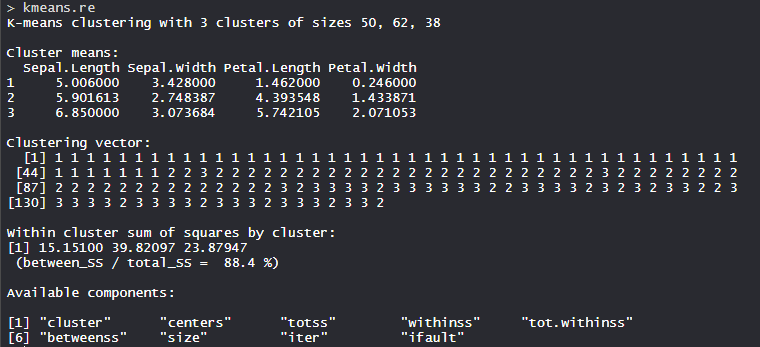The 3 clusters are made which are of 50, 62, and 38 sizes respectively. Within the cluster, the sum of squares is 88.4%.

• Cluster identification: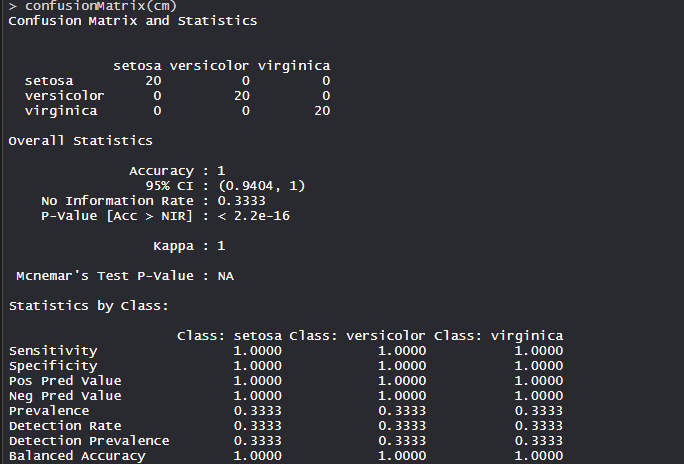The model achieved an accuracy of 100% with a p-value of less than 1. This indicates the model is good.

• Confusion Matrix: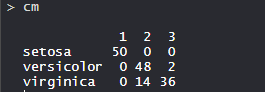So, 50 Setosa are correctly classified as Setosa. Out of 62 Versicolor, 48 Versicolor are correctly classified as Versicolor and 14 are classified as virginica. Out of 36 virginica, 19 virginica are correctly classified as virginica and 2 are classified as Versicolor.

• K-means with 3 clusters plot: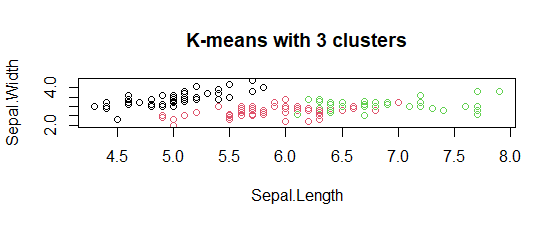The model showed 3 cluster plots with three different colors and with Sepal.length and with Sepal.width.

• Plotting cluster centers: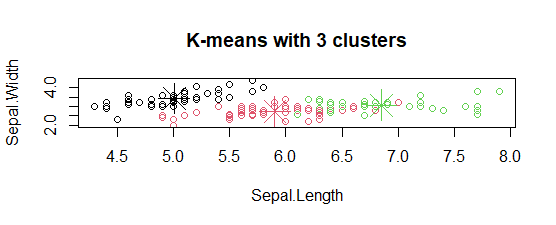In the plot, centers of clusters are marked with cross signs with the same color of the cluster.

• Plot of clusters: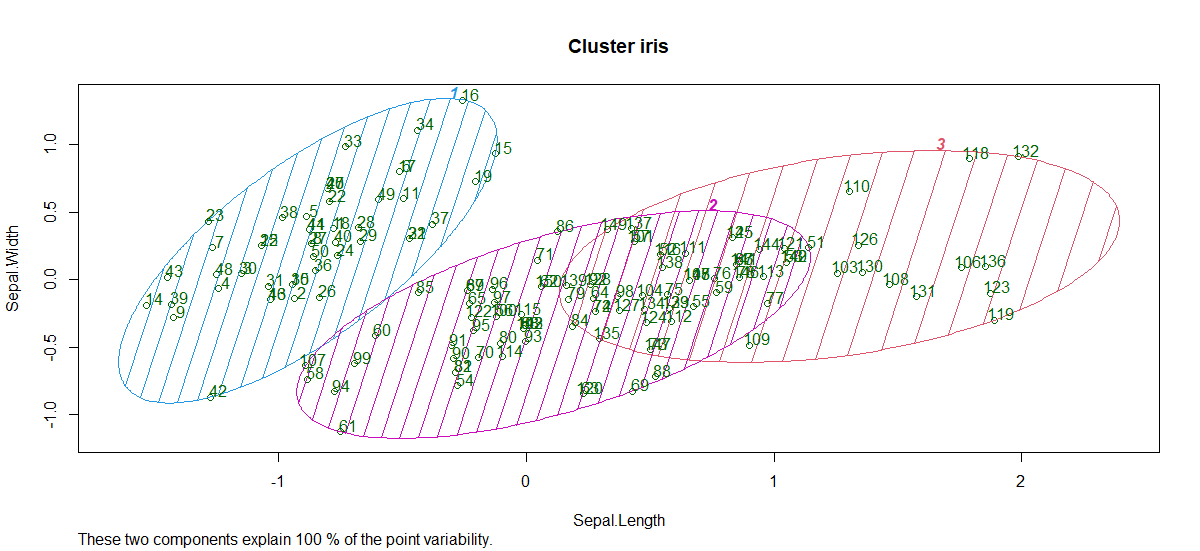So, 3 clusters are formed with varying sepal length and sepal width. Hence, the K-Means clustering algorithm is widely used in the industry.

My Personal Notes arrow_drop_upCheck out this Author's contributed articles.

If you like GeeksforGeeks and would like to contribute, you can also write an article using contribute.geeksforgeeks.org or mail your article to contribute@geeksforgeeks.org. See your article appearing on the GeeksforGeeks main page and help other Geeks.

Please Improve this article if you find anything incorrect by clicking on the "Improve Article" button below.